# TS Polycet (Polytechnic) 2019 Previous Question Paper with Answers And Model Papers With Complete AnalysisTS Polycet (Polytechnic) 2019 Previous Question Paper with Answers And Model Papers With Complete Analysis
TS Polycet (Polytechnic) Previous Year Question Papers And Model Papers:
While preparing for TS Polycet (Polytechnic), candidates must also refer to the previous year question papers of the same. Scoring well in TS Polycet (Polytechnic) and understanding weaknesses and strengths in the respective sections.

TS Polycet (Polytechnic) Previous Year Question Papers can be found on this page in PDF format. Students taking the exam to get into some of the best Polytechnic colleges/institutes in the state of Andhra Pradesh may practice these papers to get a clear idea of the structure of the exam, marking scheme, important topics, etc.

## Section — I MATHEMATICS

Q). The HCF of 96 and 72
A) 96
B) 72
C) 24
D) None

C) 24
Explanation: 96 = 2 x2x2x2x2x3
72 = 2x2x2x3x3
∴HCF of 96 and 72 = 2 x 2 x 2 x 3 = 24

Q). The LCM of 10, 20, 30
A) 10
B) 20
C) 30
D) None

D) None
Explanation: ∴L.C.M = 2 x 5 x 1 x 2 x 3 = 60

Q). The HCF of 23 x 33 x 52 and 22 x 3 x 53 is
A) 23x3x52
B) 23x33x5
C) 22 x 3 x 52
D) None

C) 22 x 3 x 52
Explanation: H.C.F=22 x 3 x 52
= 4 x 3 x 25 = 300

Q). The value of loge√e
A) 0
B) 1
C) e
D) 1/2

D) 1/2
Explanation: loge√e = loge e1/2 = 1/2 logee = ½ (1) = 1/2

Q). logya x logxy =
A) logay
B) logxa
C) logax
D) logya

B) logxa
Explanation: logya x logxy = logxa

Q). If A =(1, 2,3, 4, 5) and B = {4, 5, 6},- then find A⋂B.
A) A
B) B
C) {4,5}
D) {1,2,3}

C) {4,5}
Explanation: A ={1,2, 3, 4, 5},B = {4, 5, 6)
A ⋂ B = {1, 2, 3, 4, 5} ⋂ {4, 5, 6) = {4, 5}

Q). The cardinal number of set A = {1, 2, 4) is
A) 1
B) 4
C) 2
D) 3

D) 3
Explanation: A ={1,2,4} =>n (A) = 3

Q). The zeroes of quadratic polynomial x2 – 2x – 8 is
A) 4,-2
B) 2,8
C) 1,-2
D) 1,-8

A) 4,-2
Explanation: To find zeroes
x2-2x-8 = 0
x2 – 4x + 2x – 8 = 0
x(x-4) + 2 (x-4) = 0
x – 4 = 0 or x + 2 = 0
x = 4 or x = -2

Q). If x3 – 3 x2 + 4x + k is exactly divisible by x-2, then k =
A) 4
B) -4
C) 0
D) 1

B) -4
Explanation: f(x) = x3 -3 x2 + 4x + k
f(2) = 0
=> (2)3 – 3(2)2 + 4(2) + k= 0
=>8-12 + 8 + k = 0
=> k + 4 = 0 => k = —4

Q). Solution of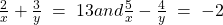A) (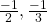)
B) (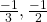)
C) (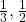)
D) (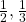)

D) ()
Explanation:= 4+9 = 13
=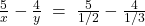= 10-12 = -2